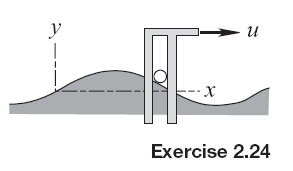### Create an Account

Already have account?

### Forgot Your Password ?

Home / Questions / The diagram shows a small ball that is pushed in the vertical plane along a hill whose ele...

# The diagram shows a small ball that is pushed in the vertical plane along a hill whose elevation is

The diagram shows a small ball that is pushed in the vertical plane along a hill whose elevation  is y = Hs in (πx/L) . The motion is actuated by an angle arm that translates horizontally at constant speed u. It may be assumed that the ball remains in contact with the hill.

(a) Derive expressions for the velocity and acceleration of the ball as functions of its horizontal distance x from the origin.

(b) Determine the maximum speed v of the ball and the value(s) of x at which it occurs.

(c) Determine the maximum acceleration magnitude of the ball and the value(s) of x at which it occurs. (d) What is the largest value of u for which the ball will remain in contact with the hill whenx = L/2? Friction is negligible, but gravity is not.Jun 18 2020 View more View Less

#### Answer (Solved)Subscribe To Get Solution